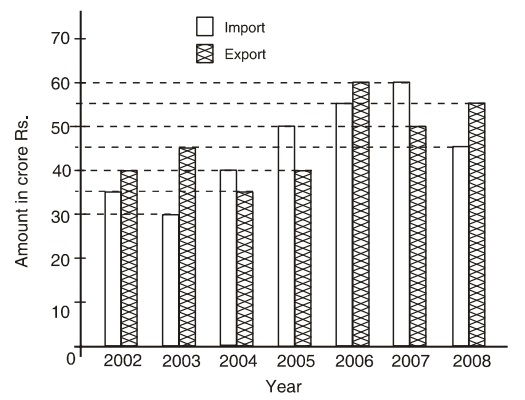Home » Data Interpretation » Bar graph » Question

#### Data Interpretation

Direction: Study the following graph carefully to answer the questions given below it.
Imports and Exports of spare parts by an automobile company over the given years.1. The total export in the years 2004, 2005 and 2008 together are what percent of the total imports during the same period?
1. 99.33
2. 93.67
3. 96.3%
4. 107.41
##### Correct Option: C

In 2004, 2005 and 2008, total import = 40 + 50 + 45 = Rs. 135 crores
In 2004, 2005 and 2008, total export = 35 + 40 + 55 = Rs. 130 crores
Required percentage = 130/135 x 100 = 96.3%